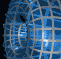Português | English Beyond 3D > A Rotation of Cubes > Artist's comments

# Artist's Comments on A Rotation of CubesThis image represents a sequence of views of a hypercube rotating in four-space. Below each is a corresponding view of a cube rotating. Looking first at these, note that they are two-dimensional shadows of a cube. Our brains easily rebuild the three-dimensional cube in our minds based on these flat pictures; our goal is to use the insight we gain from this to help imagine the hypercube in four dimensions.

Just as the three-dimensional cube casts a two-dimensional shadow, so the four-dimensional hypercube casts a three-dimensional shadow. These are the images floating above the flat planes. From these, we want to reconstruct the hypercube in our heads, just as we do the cube from the flat images below.

To do this, we need to look at the two-dimensional images and carefully note the features that we use to recognize the nature of the three-dimension cube of which it is a shadow. Then we look for the analogous features in the three-dimensional shadows. In the shadows of the cube, two opposite faces are outlined in yellow and orange; but just as the faces of a cube are two-dimensional squares, so the "faces" of the hypercube are three-dimensional cubes. In the shadows of the hypercube, two of its opposite "faces" are outlined in color as well.

Note that, in the shadow of the cube, the two colored squares of the cube often do not appear to be square, but rather trapezoids. The faces are still squares on the three-dimensional cube, but their shadows are distorted because part of the face is closer to the light source, and so has a larger shadow. The faces look square in the shadow only in one case: when the light hits the faces head on. In this case, the cube appears as a square inside a larger square; the large square represents the face closest to the light source, which has the largest shadow.

Similarly, the cubical faces of the hypercube usually have shadows that appear to be truncated pyramids rather than cubes, and they are distorted in exactly the same way that the faces of the cube are distorted. Only in one case are their shadows actually cubes: when the light source hits the two faces perpendicularly. The shadow of the face closest to the light source is the larger cube (orange); the farthest cube from the light is the inner cube (yellow).

```
```